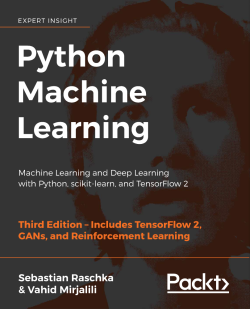# Python Machine Learning - Third Edition4.5 (45 reviews total)
By Sebastian Raschka , Vahid Mirjalili
• \$41.99 eBook
• \$51.99 Print + eBook
• \$12.99 eBook + Subscription
What do you get with a Packt Subscription?

• Constantly updated with 100+ new titles each month
• Breadth and depth in over 1,000+ technologies

Python Machine Learning, Third Edition is a comprehensive guide to machine learning and deep learning with Python. It acts as both a step-by-step tutorial, and a reference you'll keep coming back to as you build your machine learning systems.

Packed with clear explanations, visualizations, and working examples, the book covers all the essential machine learning techniques in depth. While some books teach you only to follow instructions, with this machine learning book, Raschka and Mirjalili teach the principles behind machine learning, allowing you to build models and applications for yourself.

Updated for TensorFlow 2.0, this new third edition introduces readers to its new Keras API features, as well as the latest additions to scikit-learn. It's also expanded to cover cutting-edge reinforcement learning techniques based on deep learning, as well as an introduction to GANs. Finally, this book also explores a subfield of natural language processing (NLP) called sentiment analysis, helping you learn how to use machine learning algorithms to classify documents.

This book is your companion to machine learning with Python, whether you're a Python developer new to machine learning or want to deepen your knowledge of the latest developments.

Publication date:
December 2019
Publisher
Packt
Pages
772
ISBN
9781789955750

# Giving Computers the Ability to Learn from Data

In my opinion, machine learning, the application and science of algorithms that make sense of data, is the most exciting field of all the computer sciences! We are living in an age where data comes in abundance; using self-learning algorithms from the field of machine learning, we can turn this data into knowledge. Thanks to the many powerful open source libraries that have been developed in recent years, there has probably never been a better time to break into the machine learning field and learn how to utilize powerful algorithms to spot patterns in data and make predictions about future events.

In this chapter, you will learn about the main concepts and different types of machine learning. Together with a basic introduction to the relevant terminology, we will lay the groundwork for successfully using machine learning techniques for practical problem solving.

In this chapter, we will cover the following topics:

• The general concepts of machine learning
• The three types of learning and basic terminology
• The building blocks for successfully designing machine learning systems
• Installing and setting up Python for data analysis and machine learning

# Building intelligent machines to transform data into knowledge

In this age of modern technology, there is one resource that we have in abundance: a large amount of structured and unstructured data. In the second half of the 20th century, machine learning evolved as a subfield of artificial intelligence (AI) involving self-learning algorithms that derive knowledge from data in order to make predictions.

Instead of requiring humans to manually derive rules and build models from analyzing large amounts of data, machine learning offers a more efficient alternative for capturing the knowledge in data to gradually improve the performance of predictive models and make data-driven decisions.

Not only is machine learning becoming increasingly important in computer science research, but it is also playing an ever-greater role in our everyday lives. Thanks to machine learning, we enjoy robust email spam filters, convenient text and voice recognition software, reliable web search engines, and challenging chess-playing programs. Hopefully soon, we will add safe and efficient self-driving cars to this list. Also, notable progress has been made in medical applications; for example, researchers demonstrated that deep learning models can detect skin cancer with near-human accuracy (https://www.nature.com/articles/nature21056). Another milestone was recently achieved by researchers at DeepMind, who used deep learning to predict 3D protein structures, outperforming physics-based approaches for the first time (https://deepmind.com/blog/alphafold/).

# The three different types of machine learning

In this section, we will take a look at the three types of machine learning: supervised learning, unsupervised learning, and reinforcement learning. We will learn about the fundamental differences between the three different learning types and, using conceptual examples, we will develop an understanding of the practical problem domains where they can be applied:

## Making predictions about the future with supervised learning

The main goal in supervised learning is to learn a model from labeled training data that allows us to make predictions about unseen or future data. Here, the term "supervised" refers to a set of training examples (data inputs) where the desired output signals (labels) are already known. The following figure summarizes a typical supervised learning workflow, where the labeled training data is passed to a machine learning algorithm for fitting a predictive model that can make predictions on new, unlabeled data inputs:

Considering the example of email spam filtering, we can train a model using a supervised machine learning algorithm on a corpus of labeled emails, which are correctly marked as spam or non-spam, to predict whether a new email belongs to either of the two categories. A supervised learning task with discrete class labels, such as in the previous email spam filtering example, is also called a classification task. Another subcategory of supervised learning is regression, where the outcome signal is a continuous value.

### Classification for predicting class labels

Classification is a subcategory of supervised learning where the goal is to predict the categorical class labels of new instances, based on past observations. Those class labels are discrete, unordered values that can be understood as the group memberships of the instances. The previously mentioned example of email spam detection represents a typical example of a binary classification task, where the machine learning algorithm learns a set of rules in order to distinguish between two possible classes: spam and non-spam emails.

The following figure illustrates the concept of a binary classification task given 30 training examples; 15 training examples are labeled as the negative class (minus signs) and 15 training examples are labeled as the positive class (plus signs). In this scenario, our dataset is two-dimensional, which means that each example has two values associated with it: x1 and x2. Now, we can use a supervised machine learning algorithm to learn a rule—the decision boundary represented as a dashed line—that can separate those two classes and classify new data into each of those two categories given its x1 and x2 values:

However, the set of class labels does not have to be of a binary nature. The predictive model learned by a supervised learning algorithm can assign any class label that was presented in the training dataset to a new, unlabeled instance.

A typical example of a multiclass classification task is handwritten character recognition. We can collect a training dataset that consists of multiple handwritten examples of each letter in the alphabet. The letters ("A," "B," "C," and so on) will represent the different unordered categories or class labels that we want to predict. Now, if a user provides a new handwritten character via an input device, our predictive model will be able to predict the correct letter in the alphabet with certain accuracy. However, our machine learning system will be unable to correctly recognize any of the digits between 0 and 9, for example, if they were not part of the training dataset.

### Regression for predicting continuous outcomes

We learned in the previous section that the task of classification is to assign categorical, unordered labels to instances. A second type of supervised learning is the prediction of continuous outcomes, which is also called regression analysis. In regression analysis, we are given a number of predictor (explanatory) variables and a continuous response variable (outcome), and we try to find a relationship between those variables that allows us to predict an outcome.

Note that in the field of machine learning, the predictor variables are commonly called "features," and the response variables are usually referred to as "target variables." We will adopt these conventions throughout this book.

For example, let's assume that we are interested in predicting the math SAT scores of students. If there is a relationship between the time spent studying for the test and the final scores, we could use it as training data to learn a model that uses the study time to predict the test scores of future students who are planning to take this test.

Regression toward the mean

The term "regression" was devised by Francis Galton in his article Regression towards Mediocrity in Hereditary Stature in 1886. Galton described the biological phenomenon that the variance of height in a population does not increase over time.

He observed that the height of parents is not passed on to their children, but instead, their children's height regresses toward the population mean.

The following figure illustrates the concept of linear regression. Given a feature variable, x, and a target variable, y, we fit a straight line to this data that minimizes the distance—most commonly the average squared distance—between the data points and the fitted line. We can now use the intercept and slope learned from this data to predict the target variable of new data:

## Solving interactive problems with reinforcement learning

Another type of machine learning is reinforcement learning. In reinforcement learning, the goal is to develop a system (agent) that improves its performance based on interactions with the environment. Since the information about the current state of the environment typically also includes a so-called reward signal, we can think of reinforcement learning as a field related to supervised learning. However, in reinforcement learning, this feedback is not the correct ground truth label or value, but a measure of how well the action was measured by a reward function. Through its interaction with the environment, an agent can then use reinforcement learning to learn a series of actions that maximizes this reward via an exploratory trial-and-error approach or deliberative planning.

A popular example of reinforcement learning is a chess engine. Here, the agent decides upon a series of moves depending on the state of the board (the environment), and the reward can be defined as win or lose at the end of the game:

There are many different subtypes of reinforcement learning. However, a general scheme is that the agent in reinforcement learning tries to maximize the reward through a series of interactions with the environment. Each state can be associated with a positive or negative reward, and a reward can be defined as accomplishing an overall goal, such as winning or losing a game of chess. For instance, in chess, the outcome of each move can be thought of as a different state of the environment.

To explore the chess example further, let's think of visiting certain configurations on the chess board as being associated with states that will more likely lead to winning—for instance, removing an opponent's chess piece from the board or threatening the queen. Other positions, however, are associated with states that will more likely result in losing the game, such as losing a chess piece to the opponent in the following turn. Now, in the game of chess, the reward (either positive for winning or negative for losing the game) will not be given until the end of the game. In addition, the final reward will also depend on how the opponent plays. For example, the opponent may sacrifice the queen but eventually win the game.

Reinforcement learning is concerned with learning to choose a series of actions that maximizes the total reward, which could be earned either immediately after taking an action or via delayed feedback.

## Discovering hidden structures with unsupervised learning

In supervised learning, we know the right answer beforehand when we train a model, and in reinforcement learning, we define a measure of reward for particular actions carried out by the agent. In unsupervised learning, however, we are dealing with unlabeled data or data of unknown structure. Using unsupervised learning techniques, we are able to explore the structure of our data to extract meaningful information without the guidance of a known outcome variable or reward function.

### Finding subgroups with clustering

Clustering is an exploratory data analysis technique that allows us to organize a pile of information into meaningful subgroups (clusters) without having any prior knowledge of their group memberships. Each cluster that arises during the analysis defines a group of objects that share a certain degree of similarity but are more dissimilar to objects in other clusters, which is why clustering is also sometimes called unsupervised classification. Clustering is a great technique for structuring information and deriving meaningful relationships from data. For example, it allows marketers to discover customer groups based on their interests, in order to develop distinct marketing programs.

The following figure illustrates how clustering can be applied to organizing unlabeled data into three distinct groups based on the similarity of their features, x1 and x2:

### Dimensionality reduction for data compression

Another subfield of unsupervised learning is dimensionality reduction. Often, we are working with data of high dimensionality—each observation comes with a high number of measurements—that can present a challenge for limited storage space and the computational performance of machine learning algorithms. Unsupervised dimensionality reduction is a commonly used approach in feature preprocessing to remove noise from data, which can also degrade the predictive performance of certain algorithms, and compress the data onto a smaller dimensional subspace while retaining most of the relevant information.

Sometimes, dimensionality reduction can also be useful for visualizing data; for example, a high-dimensional feature set can be projected onto one-, two-, or three-dimensional feature spaces in order to visualize it via 2D or 3D scatterplots or histograms. The following figure shows an example where nonlinear dimensionality reduction was applied to compress a 3D Swiss Roll onto a new 2D feature subspace:

# Introduction to the basic terminology and notations

Now that we have discussed the three broad categories of machine learning—supervised, unsupervised, and reinforcement learning—let's have a look at the basic terminology that we will be using throughout this book. The following subsection covers the common terms we will be using when referring to different aspects of a dataset, as well as the mathematical notation to communicate more precisely and efficiently.

As machine learning is a vast field and very interdisciplinary, you are guaranteed to encounter many different terms that refer to the same concepts sooner rather than later. The second subsection collects many of the most commonly used terms that are found in machine learning literature, which may be useful to you as a reference section when reading more diverse machine learning literature.

## Notation and conventions used in this book

The following table depicts an excerpt of the Iris dataset, which is a classic example in the field of machine learning. The Iris dataset contains the measurements of 150 Iris flowers from three different species—Setosa, Versicolor, and Virginica. Here, each flower example represents one row in our dataset, and the flower measurements in centimeters are stored as columns, which we also call the features of the dataset:

To keep the notation and implementation simple yet efficient, we will make use of some of the basics of linear algebra. In the following chapters, we will use a matrix and vector notation to refer to our data. We will follow the common convention to represent each example as a separate row in a feature matrix, X, where each feature is stored as a separate column.

The Iris dataset, consisting of 150 examples and four features, can then be written as a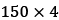matrix,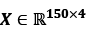:

Notational conventions

For the rest of this book, unless noted otherwise, we will use the superscript i to refer to the ith training example, and the subscript j to refer to the jth dimension of the training dataset.

We will use lowercase, bold-face letters to refer to vectors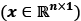and uppercase, bold-face letters to refer to matrices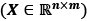. To refer to single elements in a vector or matrix, we will write the letters in italics (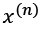or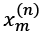, respectively).

For example,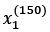refers to the first dimension of flower example 150, the sepal length. Thus, each row in this feature matrix represents one flower instance and can be written as a four-dimensional row vector,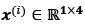:

And each feature dimension is a 150-dimensional column vector,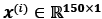. For example:

Similarly, we will store the target variables (here, class labels) as a 150-dimensional column vector:

## Machine learning terminology

Machine learning is a vast field and also very interdisciplinary as it brings together many scientists from other areas of research. As it happens, many terms and concepts have been rediscovered or redefined and may already be familiar to you but appear under different names. For your convenience, in the following list, you can find a selection of commonly used terms and their synonyms that you may find useful when reading this book and machine learning literature in general:

• Training example: A row in a table representing the dataset and synonymous with an observation, record, instance, or sample (in most contexts, sample refers to a collection of training examples).
• Training: Model fitting, for parametric models similar to parameter estimation.
• Feature, abbrev. x: A column in a data table or data (design) matrix. Synonymous with predictor, variable, input, attribute, or covariate.
• Target, abbrev. y: Synonymous with outcome, output, response variable, dependent variable, (class) label, and ground truth.
• Loss function: Often used synonymously with a cost function. Sometimes the loss function is also called an error function. In some literature, the term "loss" refers to the loss measured for a single data point, and the cost is a measurement that computes the loss (average or summed) over the entire dataset.

# A roadmap for building machine learning systems

In previous sections, we discussed the basic concepts of machine learning and the three different types of learning. In this section, we will discuss the other important parts of a machine learning system accompanying the learning algorithm.

The following diagram shows a typical workflow for using machine learning in predictive modeling, which we will discuss in the following subsections:

## Preprocessing – getting data into shape

Let's begin with discussing the roadmap for building machine learning systems. Raw data rarely comes in the form and shape that is necessary for the optimal performance of a learning algorithm. Thus, the preprocessing of the data is one of the most crucial steps in any machine learning application.

If we take the Iris flower dataset from the previous section as an example, we can think of the raw data as a series of flower images from which we want to extract meaningful features. Useful features could be the color, hue, and intensity of the flowers, or the height, length, and width of the flowers.

Many machine learning algorithms also require that the selected features are on the same scale for optimal performance, which is often achieved by transforming the features in the range [0, 1] or a standard normal distribution with zero mean and unit variance, as we will see in later chapters.

Some of the selected features may be highly correlated and therefore redundant to a certain degree. In those cases, dimensionality reduction techniques are useful for compressing the features onto a lower dimensional subspace. Reducing the dimensionality of our feature space has the advantage that less storage space is required, and the learning algorithm can run much faster. In certain cases, dimensionality reduction can also improve the predictive performance of a model if the dataset contains a large number of irrelevant features (or noise); that is, if the dataset has a low signal-to-noise ratio.

To determine whether our machine learning algorithm not only performs well on the training dataset but also generalizes well to new data, we also want to randomly divide the dataset into a separate training and test dataset. We use the training dataset to train and optimize our machine learning model, while we keep the test dataset until the very end to evaluate the final model.

## Training and selecting a predictive model

As you will see in later chapters, many different machine learning algorithms have been developed to solve different problem tasks. An important point that can be summarized from David Wolpert's famous No free lunch theorems is that we can't get learning "for free" (The Lack of A Priori Distinctions Between Learning Algorithms, D.H. Wolpert, 1996; No free lunch theorems for optimization, D.H. Wolpert and W.G. Macready, 1997). We can relate this concept to the popular saying, "I suppose it is tempting, if the only tool you have is a hammer, to treat everything as if it were a nail" (Abraham Maslow, 1966). For example, each classification algorithm has its inherent biases, and no single classification model enjoys superiority if we don't make any assumptions about the task. In practice, it is therefore essential to compare at least a handful of different algorithms in order to train and select the best performing model. But before we can compare different models, we first have to decide upon a metric to measure performance. One commonly used metric is classification accuracy, which is defined as the proportion of correctly classified instances.

One legitimate question to ask is this: how do we know which model performs well on the final test dataset and real-world data if we don't use this test dataset for the model selection, but keep it for the final model evaluation? In order to address the issue embedded in this question, different techniques summarized as "cross-validation" can be used. In cross-validation, we further divide a dataset into training and validation subsets in order to estimate the generalization performance of the model. Finally, we also cannot expect that the default parameters of the different learning algorithms provided by software libraries are optimal for our specific problem task. Therefore, we will make frequent use of hyperparameter optimization techniques that help us to fine-tune the performance of our model in later chapters.

We can think of those hyperparameters as parameters that are not learned from the data but represent the knobs of a model that we can turn to improve its performance. This will become much clearer in later chapters when we see actual examples.

## Evaluating models and predicting unseen data instances

After we have selected a model that has been fitted on the training dataset, we can use the test dataset to estimate how well it performs on this unseen data to estimate the so-called generalization error. If we are satisfied with its performance, we can now use this model to predict new, future data. It is important to note that the parameters for the previously mentioned procedures, such as feature scaling and dimensionality reduction, are solely obtained from the training dataset, and the same parameters are later reapplied to transform the test dataset, as well as any new data instances—the performance measured on the test data may be overly optimistic otherwise.

# Using Python for machine learning

Python is one of the most popular programming languages for data science and thanks to its very active developer and open source community, a large number of useful libraries for scientific computing and machine learning have been developed.

Although the performance of interpreted languages, such as Python, for computation-intensive tasks is inferior to lower-level programming languages, extension libraries such as NumPy and SciPy have been developed that build upon lower-layer Fortran and C implementations for fast vectorized operations on multidimensional arrays.

For machine learning programming tasks, we will mostly refer to the scikit-learn library, which is currently one of the most popular and accessible open source machine learning libraries. In the later chapters, when we focus on a subfield of machine learning called deep learning, we will use the latest version of the TensorFlow library, which specializes in training so-called deep neural network models very efficiently by utilizing graphics cards.

## Installing Python and packages from the Python Package Index

Python is available for all three major operating systems—Microsoft Windows, macOS, and Linux—and the installer, as well as the documentation, can be downloaded from the official Python website: https://www.python.org.

This book is written for Python version 3.7 or higher, and it is recommended that you use the most recent version of Python 3 that is currently available. Some of the code may also be compatible with Python 2.7, but as the official support for Python 2.7 ends in 2019, and the majority of open source libraries have already stopped supporting Python 2.7 (https://python3statement.org), we strongly advise that you use Python 3.7 or newer.

The additional packages that we will be using throughout this book can be installed via the `pip` installer program, which has been part of the Python Standard Library since Python 3.3. More information about `pip` can be found at https://docs.python.org/3/installing/index.html.

After we have successfully installed Python, we can execute pip from the terminal to install additional Python packages:

``````pip install SomePackage
``````

Already installed packages can be updated via the `--upgrade` flag:

``````pip install SomePackage --upgrade
``````

## Using the Anaconda Python distribution and package manager

A highly recommended alternative Python distribution for scientific computing is Anaconda by Continuum Analytics. Anaconda is a free—including commercial use—enterprise-ready Python distribution that bundles all the essential Python packages for data science, math, and engineering into one user-friendly, cross-platform distribution. The Anaconda installer can be downloaded at https://docs.anaconda.com/anaconda/install/, and an Anaconda quick start guide is available at https://docs.anaconda.com/anaconda/user-guide/getting-started/.

After successfully installing Anaconda, we can install new Python packages using the following command:

``````conda install SomePackage
``````

Existing packages can be updated using the following command:

``````conda update SomePackage
``````

## Packages for scientific computing, data science, and machine learning

Throughout this book, we will mainly use NumPy's multidimensional arrays to store and manipulate data. Occasionally, we will make use of pandas, which is a library built on top of NumPy that provides additional higher-level data manipulation tools that make working with tabular data even more convenient. To augment your learning experience and visualize quantitative data, which is often extremely useful to make sense of it, we will use the very customizable Matplotlib library.

The version numbers of the major Python packages that were used to write this book are mentioned in the following list. Please make sure that the version numbers of your installed packages are equal to, or greater than, these version numbers to ensure that the code examples run correctly:

• NumPy 1.17.4
• SciPy 1.3.1
• scikit-learn 0.22.0
• Matplotlib 3.1.0
• pandas 0.25.3

# Summary

In this chapter, we explored machine learning at a very high level and familiarized ourselves with the big picture and major concepts that we are going to explore in the following chapters in more detail. We learned that supervised learning is composed of two important subfields: classification and regression. While classification models allow us to categorize objects into known classes, we can use regression analysis to predict the continuous outcomes of target variables. Unsupervised learning not only offers useful techniques for discovering structures in unlabeled data, but it can also be useful for data compression in feature preprocessing steps.

We briefly went over the typical roadmap for applying machine learning to problem tasks, which we will use as a foundation for deeper discussions and hands-on examples in the following chapters. Finally, we set up our Python environment and installed and updated the required packages to get ready to see machine learning in action.

Later in this book, in addition to machine learning itself, we will introduce different techniques to preprocess a dataset, which will help you to get the best performance out of different machine learning algorithms. While we will cover classification algorithms quite extensively throughout the book, we will also explore different techniques for regression analysis and clustering.

We have an exciting journey ahead, covering many powerful techniques in the vast field of machine learning. However, we will approach machine learning one step at a time, building upon our knowledge gradually throughout the chapters of this book. In the following chapter, we will start this journey by implementing one of the earliest machine learning algorithms for classification, which will prepare us for Chapter 3, A Tour of Machine Learning Classifiers Using scikit-learn, where we will cover more advanced machine learning algorithms using the scikit-learn open source machine learning library.

• Sebastian Raschka

Sebastian Raschka is an Assistant Professor of Statistics at the University of Wisconsin-Madison focusing on machine learning and deep learning research. As Lead AI Educator at Grid AI, Sebastian plans to continue following his passion for helping people get into machine learning and artificial intelligence.

Browse publications by this author
• Vahid Mirjalili

Vahid Mirjalili is a deep learning researcher focusing on CV applications. Vahid received a Ph.D. degree in both Mechanical Engineering and Computer Science from Michigan State University.

Browse publications by this author
Latest Reviews (45 reviews total)
Gid innføring i et viktig emne
My experience has been excellent, which has focused on NumPy, Matplotlib, and pandas, and have introduced deep learning concepts, but could be improved to cover both PyTorch and TensorFlow more in depth using concrete examples
It is a topic that interest me greatly.